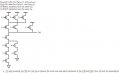# Transistor Sizing

#### thisonedude

Joined Apr 20, 2014
52
Hi everyone. I'm currently working on the following problemSo i am having trouble understanding what it means by worst-case equivalent resistance. I understand the following: The worst case path for the PUN is when only on path exists between the Output and Vdd. This occurs when EFGHIJ = 011011. For the PDN the worst case occurs again when only one path (longest) exists between the output and GND. Int his case it occurs when EFGHI = 111001. Okay so i understand this much. I also know that transistors in series are added and in parallel are halfed. so EF for PDN would be 2R and HI for PUN would be R/2 as an example. but how do i calculate the worst case resistance? How do i calculate the Width? I'm looking more to understand what it is asking me to find, than the final answer. how do i approach this problem?

Last edited:

#### WBahn

Joined Mar 31, 2012
29,521
Does it tell you what Lmin is?

#### thisonedude

Joined Apr 20, 2014
52
Does it tell you what Lmin is?
All that is given in the problem is attached to the picture. Lmin is equal to Lp. and the ratio between the width and length is 1.

#### WBahn

Joined Mar 31, 2012
29,521
What the picture tells me is that the resistance figures given are for minimum-length square transistors. But when the problem then states that Wn = 195 nm and that you are supposed to find Wp, that implies that the transistors used in the problem are not minimum-length square transistors.

But that should be okay because you are not asked to find the actual resistance, just to balance them.

So, assume that the transistors are all minimum-length square transistors and find the ratio of the worst case pull-up to the worse case pull-down resistance. What will that tell you about the needed ratio of Wp/Wn in order to make them equal?

#### thisonedude

Joined Apr 20, 2014
52
What the picture tells me is that the resistance figures given are for minimum-length square transistors. But when the problem then states that Wn = 195 nm and that you are supposed to find Wp, that implies that the transistors used in the problem are not minimum-length square transistors.

But that should be okay because you are not asked to find the actual resistance, just to balance them.

So, assume that the transistors are all minimum-length square transistors and find the ratio of the worst case pull-up to the worse case pull-down resistance. What will that tell you about the needed ratio of Wp/Wn in order to make them equal?
I'm still confused but let me see if i understand this. So, the worst case pull-down would be, lets say if i take the worst path to be JEFG to Ground. This means that means i have 4Reqn. so if the transistor width Wp=195nm, then 4reqn = Reqn/4 -> Reqn = 195/8 ?

Does this mean that the ratio of Wp/Wn needs to be 1 in order to make them equal?

#### WBahn

Joined Mar 31, 2012
29,521
Nope.

The worst case resistance to ground is Rwcn = 4*Reqn.

What is the worst case resistance to Vdd, Rwcp, (in terms of Reqp)?

#### thisonedude

Joined Apr 20, 2014
52
Nope.

The worst case resistance to ground is Rwcn = 4*Reqn.

What is the worst case resistance to Vdd, Rwcp, (in terms of Reqp)?
Ohh alright so I was right about my worst case for the pull down being 4Reqn? For the pullup the worst happens when I take either EH or FI or GI. So would it be Rwcp=Reqp/2?

#### WBahn

Joined Mar 31, 2012
29,521
Ohh alright so I was right about my worst case for the pull down being 4Reqn? For the pullup the worst happens when I take either EH or FI or GI. So would it be Rwcp=Reqp/2?
If k equal NFET resistances in series are k-times one NFET resistance, why would k equal PFET resistances in series be one PFET resistance divided by k?

Does that make sense to YOU?

#### thisonedude

Joined Apr 20, 2014
52
If k equal NFET resistances in series are k-times one NFET resistance, why would k equal PFET resistances in series be one PFET resistance divided by k?

Does that make sense to YOU?
Ohhhhhhhhh yes sorry my mistake yes. Scratch my last response. Rwcp =2Reqp, since they are in series. Likewise worst case for the pull down would be the longest single path, JEFG: Rwcn=4Reqn since again they are in series. Alright but then how do I balance them? So you say that the ratio Wp/Wn would need to be one in order to make them equal?

#### thisonedude

Joined Apr 20, 2014
52
Would I have to match the equivalent worst case resistances to get the balanced case width for the Pmos

#### WBahn

Joined Mar 31, 2012
29,521
Ohhhhhhhhh yes sorry my mistake yes. Scratch my last response. Rwcp =2Reqp, since they are in series. Likewise worst case for the pull down would be the longest single path, JEFG: Rwcn=4Reqn since again they are in series. Alright but then how do I balance them? So you say that the ratio Wp/Wn would need to be one in order to make them equal?
Where did I say that the Wp/Wn ratio needed to be one? YOU said that. I asked you what it NEEDED to be in order to make the resistances the same.

So set Rwcn equal to Rwcp. Then determine what Reqp/Reqn needs to be. Then determine what Wp/Wn needs to be.